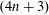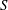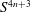Hostname: page-component-546b4f848f-fhndm Total loading time: 0 Render date: 2023-06-05T00:21:18.279Z Has data issue: false Feature Flags: { "useRatesEcommerce": true } hasContentIssue false

# Homogeneous Einstein Finsler Metrics on$(4n+3)$-dimensional Spheres

Published online by Cambridge University Press:  09 November 2018

## Abstract

In this paper, we study a class of homogeneous Finsler metrics of vanishing$S$-curvature on a$(4n+3)$-dimensional sphere. We find a second order ordinary differential equation that characterizes Einstein metrics with constant Ricci curvature$1$ in this class. Using this equation we show that there are infinitely many homogeneous Einstein metrics on$S^{4n+3}$ of constant Ricci curvature$1$ and vanishing$S$-curvature. They contain the canonical metric on$S^{4n+3}$ of constant sectional curvature$1$ and the Einstein metric of non-constant sectional curvature given by Jensen in 1973.

## MSC classification

Type
Article
Information
Canadian Mathematical Bulletin , September 2019 , pp. 509 - 523

## Access options

Get access to the full version of this content by using one of the access options below. (Log in options will check for institutional or personal access. Content may require purchase if you do not have access.)

## Footnotes

Author Mo is the corresponding author. Author Huang was supported by the National Natural Science Foundation of China 11301283 and 11571185. Mo was supported by the National Natural Science Foundation of China 11771020.

## References

Bao, D. and Robles, C., Ricci and flag curvature . In: A Sampler of Riemann-Finsler geometry , Math. Sci. Res. Inst. Publ., 50, Cambridge University Press, 2004.Google Scholar
Cheng, X., Shen, Z., and Tian, Y., A class of Einstein (𝛼, 𝛽)-metrics . Israel J. Math. 192(2012), 221249. https://doi.org/10.1007/s11856-012-0036-x.CrossRefGoogle Scholar
Chern, S. S., Finsler geometry is just Riemannian geometry without the quadratic restriction . Notices Amer. Math. Soc. 43(1996), 959963.Google Scholar
Deng, S. and Hou, Z., Invariant Finsler metrics on homogeneous manifolds . J. Phys. A 37(2004), n. 34, 82458253. https://doi.org/10.1088/0305-4470/37/34/004.CrossRefGoogle Scholar
Huang, L., Einstein Finsler metrics on S 3 with nonconstant flag curvature . Houston J. Math. 37(2011), 10711086.Google Scholar
Huang, L., Ricci curvature of left invariant Finsler metrics on Lie groups . Israel J. Math. 207(2015), 783792. https://doi.org/10.1007/s11856-015-1161-0.CrossRefGoogle Scholar
Huang, L., On the fundamental equations of homogeneous Finsler spaces . Diff. Geom. Appl. 40(2015), 187208. https://doi.org/10.1016/j.difgeo.2014.12.009.CrossRefGoogle Scholar
Huang, L., Flag curvatures of homogeneous Finsler spaces . European J. Math. 3(2017), 10001029. https://doi.org/10.1007/s40879-017-0157-1.CrossRefGoogle Scholar
Jensen, G. R., Einstein metrics on principle fibre bundles . J. Diff. Geom. 8(1973), 599614.Google Scholar
Milnor, J., Curvatures of left invariant metrics on Lie groups . Adv. Math. 21(1976), 293329. https://doi.org/10.1016/S0001-8708(76)80002-3.CrossRefGoogle Scholar
Mo, X., On the flag curvature of a Finsler space with constant S-curvature . Houston J. Math. 31(2005), 131144.Google Scholar
Sevim, E. S., Shen, Z., and Zhao, L., On a class of Ricci flat Douglas metrics . Intern. J. Math. 23(2012), 1250046, 15pp. https://doi.org/10.1142/S0129167X12500462.CrossRefGoogle Scholar
Shen, Z., Volume comparison and its application in Riemann-Finsler geometry . Adv. Math. 128(1997), 306328. https://doi.org/10.1006/aima.1997.1630.CrossRefGoogle Scholar
Shen, Z., Lectures on Finsler geometry . World Scientific Publishing Co., Singapore, 2001. https://doi.org/10.1142/9789812811622.CrossRefGoogle Scholar
Shen, Z. and Yu, C., On a class of Einstein Finsler metrics . Internat. J. Math. 25(2014), 1450030, 18pp. https://doi.org/10.1142/S0129167X1450030X.CrossRefGoogle Scholar
Wang, H., Huang, L., and Deng, S., Homogeneous Einstein-Randers metrics on spheres . Nonlinear Anal. 74(2011), 62956301. https://doi.org/10.1016/j.na.2011.06.008.CrossRefGoogle Scholar
Ziller, W., Homogeneous Einstein metrics on spheres and projective spaces . Math. Ann. 259(1982), 351358. https://doi.org/10.1007/BF01456947.CrossRefGoogle Scholar